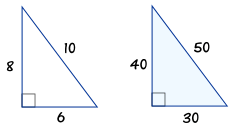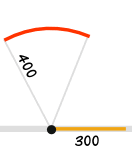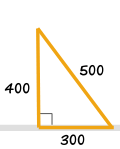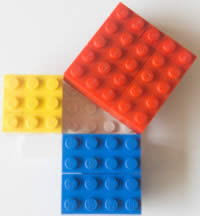# 3, 4, 5 Triangle

## Need a Right Angle (90°) Fast ... ?

 Make a 3,4,5 Triangle ! Connect three lines: 3 long 4 long 5 long And you will have a right angle (90°)## Other Lengths

You can use other lengths by multiplying each side by 2. Or by 10. Or any multiple.## Drawing It

Let us say you need to mark a right angle coming from a point on a wall.

You decide to use 300, 400 and 500 cm lines.Draw a 300 line along the wall Draw an arc 400 away from the start of the 300 line Draw an arc 500 away from the end of the 300 line Connect from the start of the 300 line to where the arcs cross And you have your "3,4,5" triangle with its right angle

## The Mathematics Behind It

The Pythagoras Theorem says:In a right-angled triangle, the square of a (a2) plus the square of b (b2) is equal to the square of c (c2): a2 + b2 = c2Let's check if it does work: 32 + 42 = 52 Calculating this becomes: 9 + 16 = 25 Yes, it works !## Other Combinations

Yes, there are other combinations that work (not just by multiplying). Here are two others:5,12,13 triangle 9,40,41 Triangle 52 + 122 = 132 92 + 402 = 412 25 + 144 = 169 (try it yourself)